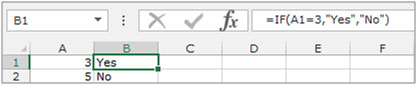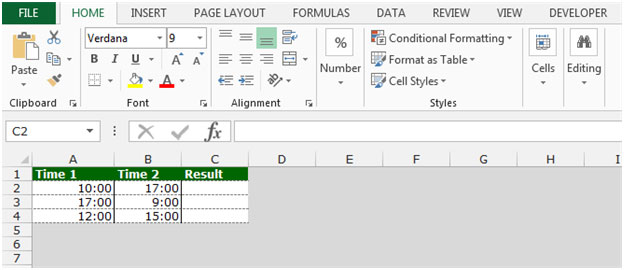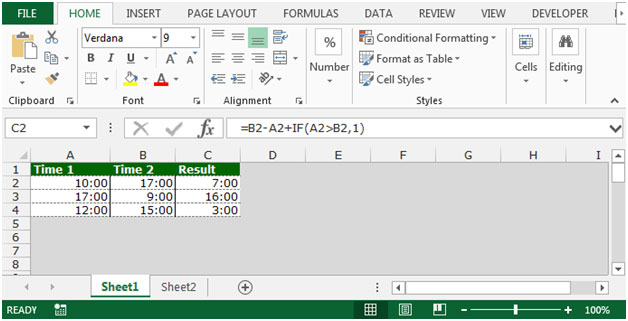# Subtracting Times in Microsoft Excel

To determine which of two values is the earliest and then Excel subtracting time  accordingly. We will use If function to create subtracting time calculator in Excel.

IF: - Check whether a condition is met and returns one value if True and another value if False.

Syntax of “IF” function =if(logical test,[value_if_true],[value_if_false])

First the formula will do the logical test, what if the output of logical test is true and false.

For example:Cell A2 and A3 contain the number 3 and 5. Ifthe number is 3, the formula should display “Yes” else “No”.

=IF (A1=3,"Yes","No")Let’s take an example to understand how we can subtract the times.

We have 2 time lists in range A1:B4 in which Column A contains Time 1 and column B contains Time 2. To find the interval between each pair of start and end time is earlier than the start time, thereby resulting in negative result.To subtract the times,follow below given steps:-

• Write the formula in cell C2.
• =B2-A2+IF(A2>B2,1)
• The function will return the time difference between two values.
• Copy the same formula and paste in the range C3:C4.This is the way we can subtract the times by using the IF function in Microsoft Excel.

1.